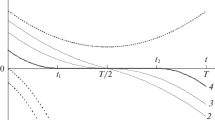# Quadratic Optimal Control in Reorienting a Spacecraft in a Fixed Time Period in a Dynamic Problem Statement

## Abstract

A dynamic problem of a spacecraft (SC) turning from an arbitrary initial angular position to the required final angular position is considered and solved. The termination time of the maneuver is known. To optimize the turn control program, the quadratic criterion of quality is used; the minimized functional characterizes the energy consumption. The construction of the optimal control of the turn is based on quaternion variables and Pontryagin’s maximum principle. It is shown that during the optimal turn, the moment of forces is parallel to a straight line immobile in space and the angular momentum direction in the rotation process of the SC is constant relative to the inertial coordinate system. The formalized equations and calculation expressions to determine the optimal turn program are obtained. For a dynamically symmetric SC, a complete solution to the reorientation problem in the closed form is given. An example and results of the mathematical simulation of the dynamics of the SC’s motion under the optimal control are presented, which demonstrate the practical feasibility of the developed method to control the SC’s attitude.

This is a preview of subscription content, log in via an institution to check access.

Price excludes VAT (USA)
Tax calculation will be finalised during checkout.

### Similar content being viewed by others### Optimal Control of the Reorientation of a Spacecraft in the Given Time with a Quadratic Performance Criterion Related to the Control and Phase Variables

M. V. LevskiiM. V. Levskii

M. V. Levskii

## References

1. K. B. Alekseev and G. G. Bebenin, Spacecraft Control (Mashinostroenie, Moscow, 1974) [in Russian].

2. N. E. Zubov, “Optimal control of terminal re-orientation of spacecraft based on algorithm with a predicting model,” Kosm. Issled. 29 (3) (1991).

3. A. I. Van’kov, “Adaptive robust control of spacecraft angular motion using predicting models,” Kosmh. Issled. 32 (4–5) (1994).

4. Yu. N. Chelnokov, “Control of spacecraft orientation by using the quaternion,” Kosm. Issled. 32 (3) (1994).

5. O. V. Ermoshina and A. P. Krishchenko, “Synthesis of programmed controls of spacecraft orientation by the method of inverse problem of dynamics,” J. Comput. Syst. Sci. Int. 39, 313 (2000).

6. M. V. Levskii, “Pontryagin’s maximum principle in optimal control problems of orientation of a spacecraft,” J. Comput. Syst. Sci. Int. 47, 974 (2008).

7. S. Liu and T. Singh, “Fuel/time optimal control of spacecraft maneuvers,” J. Guidance 20 (2) (1996).

8. S. Scrivener and R. Thompson, “Survey of time-optimal attitude maneuvers,” J. Guidance, Control Dyn. 17 (2) (1994).

9. V. N. Branets and I. P. Shmyglevskii, Application of Quaternions in Problems of Solid Body Orientation (Nauka, Moscow, 1973) [in Russian].

10. V. N. Branets, M. B. Chertok, and Yu. V. Kaznacheev, “Optimal turn of solid body with one symmetry axis,” Kosm. Issled. 22 (3) (1984).

11. H. Shen and P. Tsiotras, “Time-optimal control of axi-symmetric rigid spacecraft with two controls,” J. AIAA, Guidance, Control Dyn. 22 (5) (1999).

12. A. V. Molodenkov and Ya. G. Sapunkov, “A solution of the optimal turn problem of an axially symmetric spacecraft with bounded and pulse control under arbitrary boundary conditions,” J. Comput. Syst. Sci. Int. 46, 310 (2007).

13. F. Li and P. M. Bainum, “Numerical approach for solving rigid spacecraft minimum time attitude maneuvers,” J. Guidance, Control, Dyn. 13 (1) (1990).

14. O. V. Zelepukina and Yu. N. Chelnokov, “Construction of optimal laws of variation in the angular momentum vector of a dynamically symmetric rigid body,” Mech. Solids 46, 519 (2011).

15. A. A. Krasovskii, Automatic Flight Control Systems and their Analytical Design (Nauka, Moscow, 1973) [in Russian].

16. B. V. Raushenbakh and E. N. Tokar’, Control of Spacecraft Orientation (Nauka, Moscow, 1974) [in Russian].

17. J. L. Junkins and J. D. Turner, Optimal Spacecraft Rotational Maneuvers (Elsevier, Amsterdam, 1986).

18. R. Byers and S. Vadali, “Quasi-closed-form solution to the time-optimal rigid spacecraft reorientation problem,” J. AIAA, Guidance, Control Dyn. 16 (3) (1993).

19. M. V. Levskii, “Control of spacecraft spatial turn with minimal value of the way functional,” Actual Probl. Aviat. Aerospace Syst. 14 (1) (2009).

20. Reference Book on the Automatic Control Theory, Ed. by A. A. Krasovskii (Nauka, Moscow, 1987) [in Russian].

21. A. P. Markeev, Theoretical Mechanics (Nauka, Moscow, 1990) [in Russian].

22. L. S. Pontryagin, V. G. Boltyanskii, R. V. Gamkrelidze, and E. F. Mishchenko, Mathematical Theory of Optimal Processes (Nauka, Moscow, 1983) [in Russian].

23. G. Lastman, “A shooting method for solving two-point boundary-value problems arising from non-singular bang-bang optimal control problems,” Int. J. Control 27 (4) (1978).

24. E. Bertolazzi, F. Biral, and M. da Lio, “Symbolic-numeric efficient solution of optimal control problems for multibody systems,” J. Comput. Appl. Math. 185 (2) (2006).

25. S. Kumar, V. Kanwar, and S. Singh, “Modified efficient families of two and three-step predictor-corrector iterative methods for solving nonlinear equations,” J. Appl. Math. 1 (3) (2010).

26. T. Han and Yu. Han, “Solving large scale nonlinear equations by a new ODE numerical integration method,” J. Appl. Math. 1 (3) (2010).

27. M. V. Levskii, “Method of spacecraft turn control and the system for its realization,” RF Patent No. 2114771, Byull. Izobret. No. 19 (1998).

28. M. V. Levskii, “Control system for spacecraft spatial turnf,” RF Patent No. 2006431, Byull. Izobret. No. 2 (1994).

29. M. V. Levskii, “Device for regular rigid body precession parameters formation,” RF Patent No. 2146638, Byull. Izobret. No. 8 (2000).

## Author information

Authors

### Corresponding author

Correspondence to M. V. Levskii.

## Rights and permissions

Reprints and Permissions